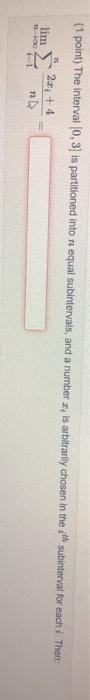### Create an Account

Already have account?

### Forgot Your Password ?

Home / Questions / (1 point) The interval (0, 3) is partitioned into n equal subintervals, and a number is ar...

# (1 point) The interval (0, 3) is partitioned into n equal subintervals, and a number is arbitrarily chosen in the ith subinterval for each i. Then 2.0 + 4

(1 point) The interval (0, 3) is partitioned into n equal subintervals, and a number is arbitrarily chosen in the ith subinterval for each i. Then 2.0 + 4Apr 15 2021 View more View Less

#### Answer (Solved)Subscribe To Get Solution